# Regents Physics Electric Fields Electric field The region

• Slides: 22Regents Physics Electric Fields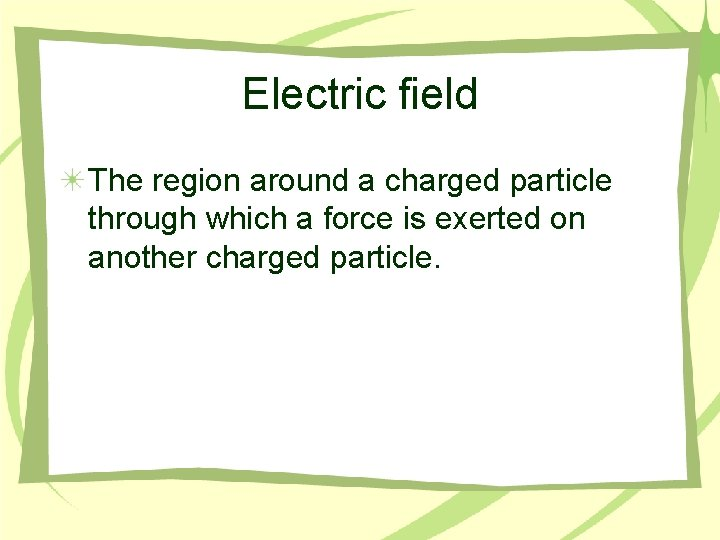Electric field The region around a charged particle through which a force is exerted on another charged particle.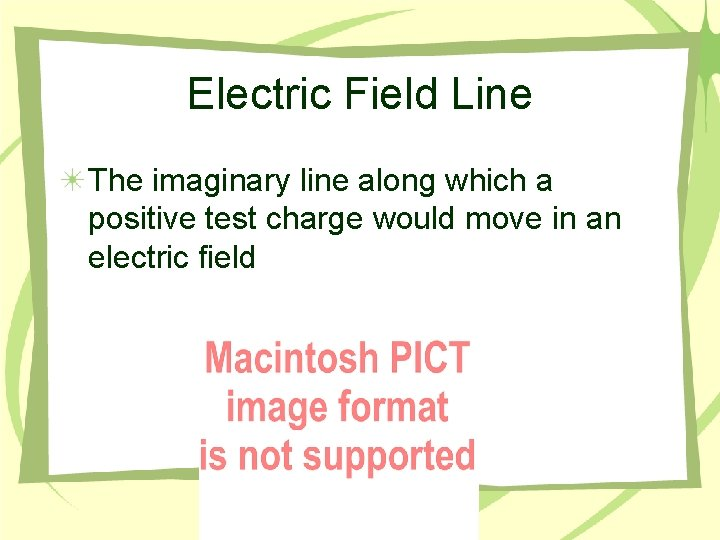Electric Field Line The imaginary line along which a positive test charge would move in an electric field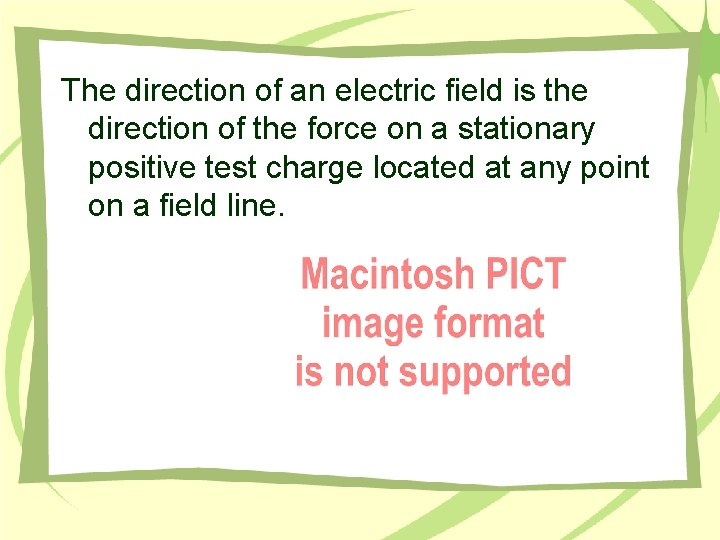The direction of an electric field is the direction of the force on a stationary positive test charge located at any point on a field line.On a curved field line, the direction of the field at any point is the tangent drawn to the field line at that point. Electric field lines begin on positive charges (or at infinity) and end on negative charges (or infinity).Field lines NEVER intersect.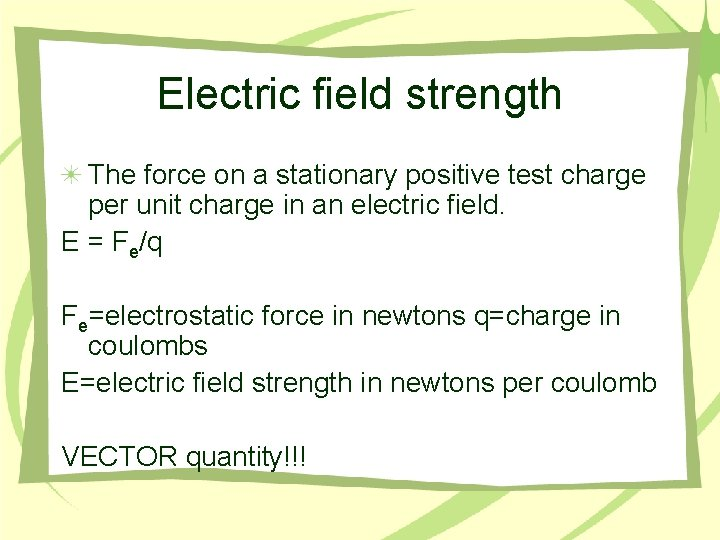Electric field strength The force on a stationary positive test charge per unit charge in an electric field. E = Fe/q Fe=electrostatic force in newtons q=charge in coulombs E=electric field strength in newtons per coulomb VECTOR quantity!!!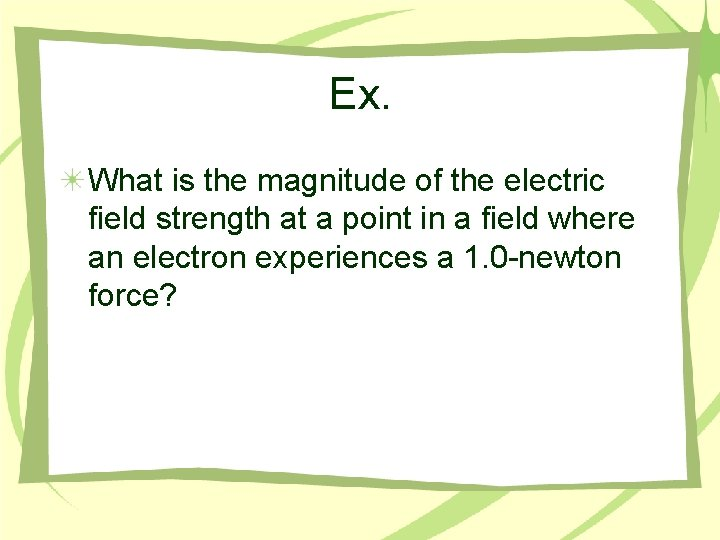Ex. What is the magnitude of the electric field strength at a point in a field where an electron experiences a 1. 0 -newton force?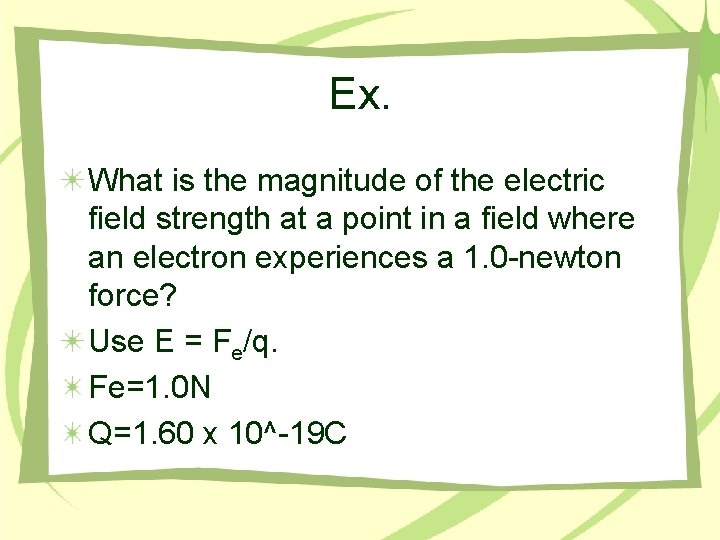Ex. What is the magnitude of the electric field strength at a point in a field where an electron experiences a 1. 0 -newton force? Use E = Fe/q. Fe=1. 0 N Q=1. 60 x 10^-19 C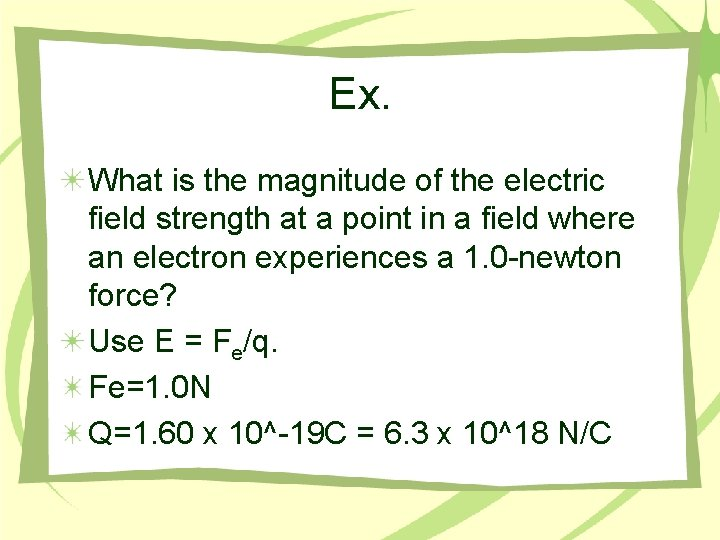Ex. What is the magnitude of the electric field strength at a point in a field where an electron experiences a 1. 0 -newton force? Use E = Fe/q. Fe=1. 0 N Q=1. 60 x 10^-19 C = 6. 3 x 10^18 N/CField Around a Point Charge or Sphere Field lines extend radially outward from a positive point charge and radially inward from a negative point charge. On a sphere, charge is distributed uniformly, and electric field lines are perpendicular to the surface.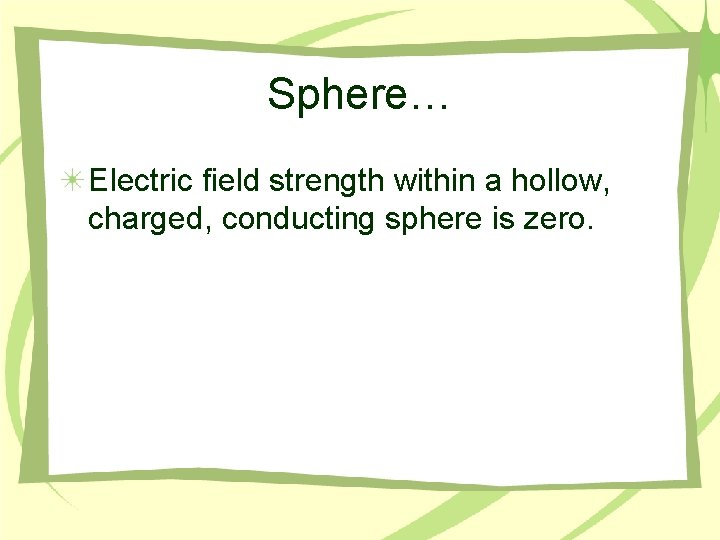Sphere… Electric field strength within a hollow, charged, conducting sphere is zero.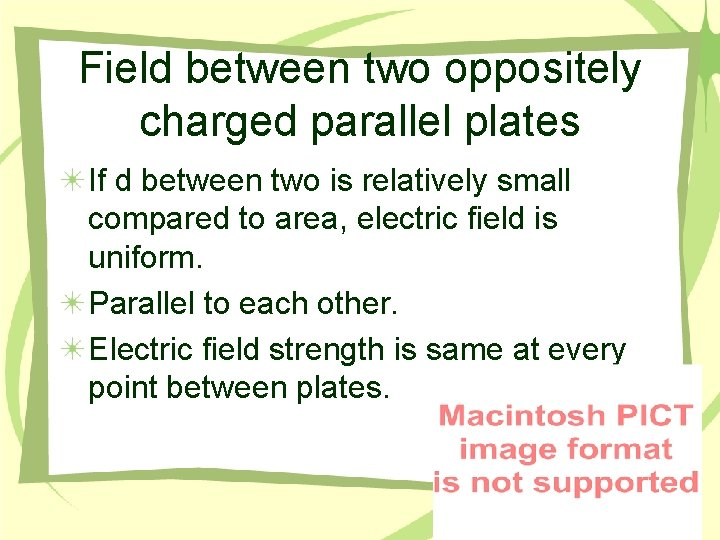Field between two oppositely charged parallel plates If d between two is relatively small compared to area, electric field is uniform. Parallel to each other. Electric field strength is same at every point between plates.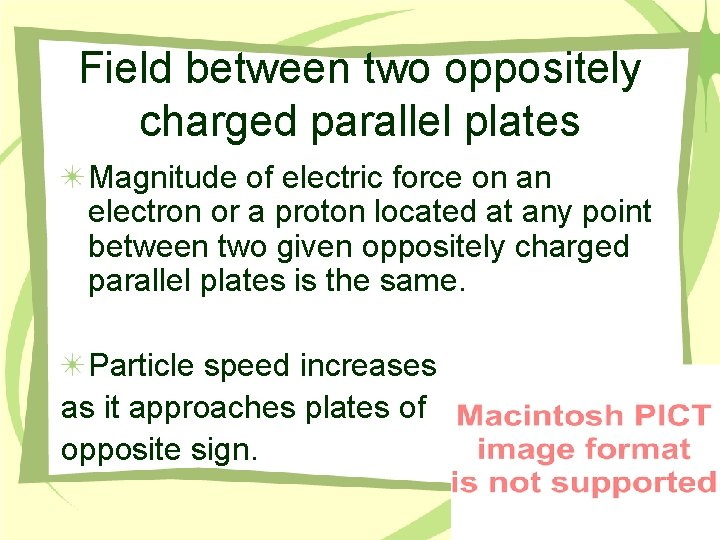Field between two oppositely charged parallel plates Magnitude of electric force on an electron or a proton located at any point between two given oppositely charged parallel plates is the same. Particle speed increases as it approaches plates of opposite sign.Potential Difference The work done (or change in potential energy) per unit charge as a charged particle is moved between two points in an electric field. V= W/q Unit= joule/coulomb = volt11/7/2008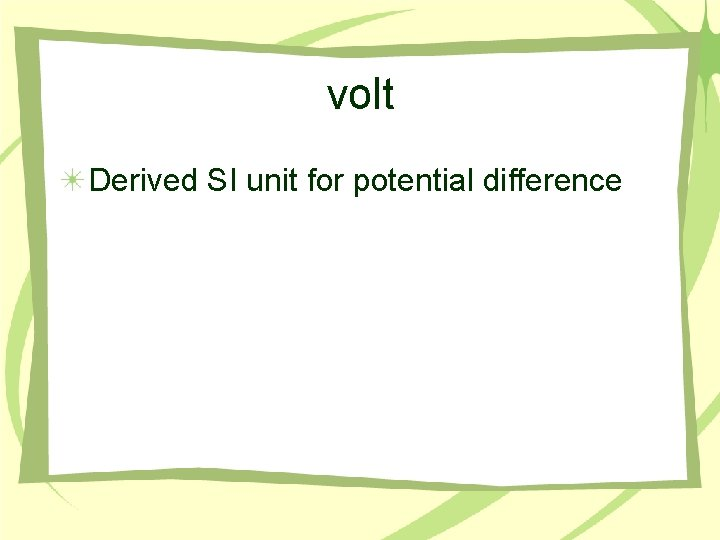volt Derived SI unit for potential difference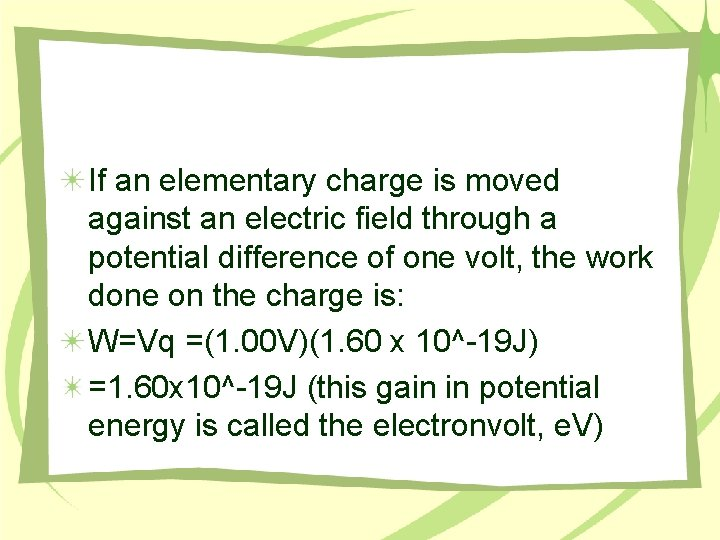If an elementary charge is moved against an electric field through a potential difference of one volt, the work done on the charge is: W=Vq =(1. 00 V)(1. 60 x 10^-19 J) =1. 60 x 10^-19 J (this gain in potential energy is called the electronvolt, e. V)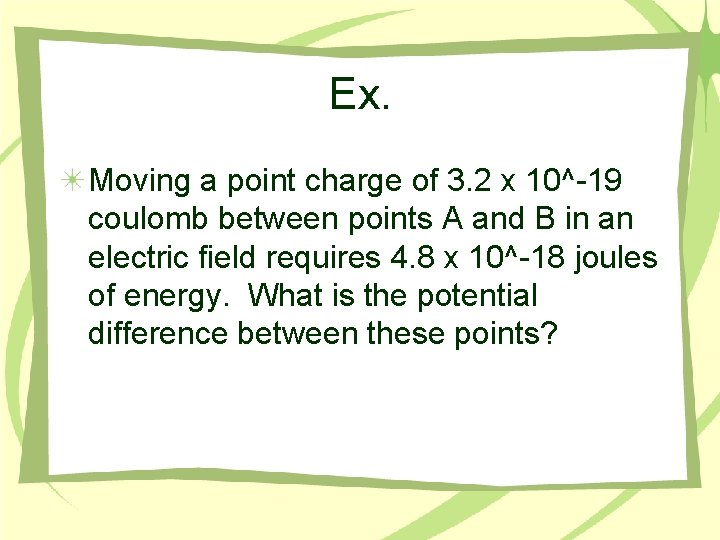Ex. Moving a point charge of 3. 2 x 10^-19 coulomb between points A and B in an electric field requires 4. 8 x 10^-18 joules of energy. What is the potential difference between these points?Ex. Moving a point charge of 3. 2 x 10^-19 coulomb between points A and B in an electric field requires 4. 8 x 10^-18 joules of energy. What is the potential difference between these points? V=W/q 4. 8 x 10^-18 J / 3. 2 x 10^-19 C =Ex. Moving a point charge of 3. 2 x 10^-19 coulomb between points A and B in an electric field requires 4. 8 x 10^-18 joules of energy. What is the potential difference between these points? V=W/q 4. 8 x 10^-18 J / 3. 2 x 10^-19 C = 15 V